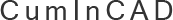CumInCAD is a Cumulative Index about publications in Computer Aided Architectural Design supported by the sibling associations ACADIA, CAADRIA, eCAADe, SIGraDi, ASCAAD and CAAD futures
 PDF papersReferences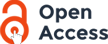authors Nievergelt, J. and Preparata, Franco P. 1982 Plane-Sweep Algorithms for Intersecting Geometric Figures Communications of the ACM. October, 1982. vol. 25: pp. 739-747 : ill. includes bibliography Algorithms in computational geometry are of increasing importance in computer-aided design, for example, in the layout of integrated circuits. The efficient computation of the intersection of several superimposed figures is a basic problem. Plane figures defined by points connected by straight line segments are considered, for example, polygons (not necessarily simple) and maps (embedded planar graphs). The regions into which the plane is partitioned by these intersecting figures are to be processed in various ways such as listing the boundary of each region in cyclic order or sweeping the interior of each region. Let m be the total number of points of all the figures involved and s be the total number of intersections of all line segments. A two plane-sweep algorithm that solves the problems above is presented; in the general case (non convexity) in time O((n+s)log-n) and space O(n+s); when the regions of each given figure are convex, the same can be achieved in time O(n log n +s) and space O(n) computational geometry, algorithms, intersection, mapping, polygons, data structures, analysis CADline Content-type: text/plain 2003/06/02 08:24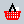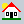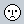CUMINCAD Papers